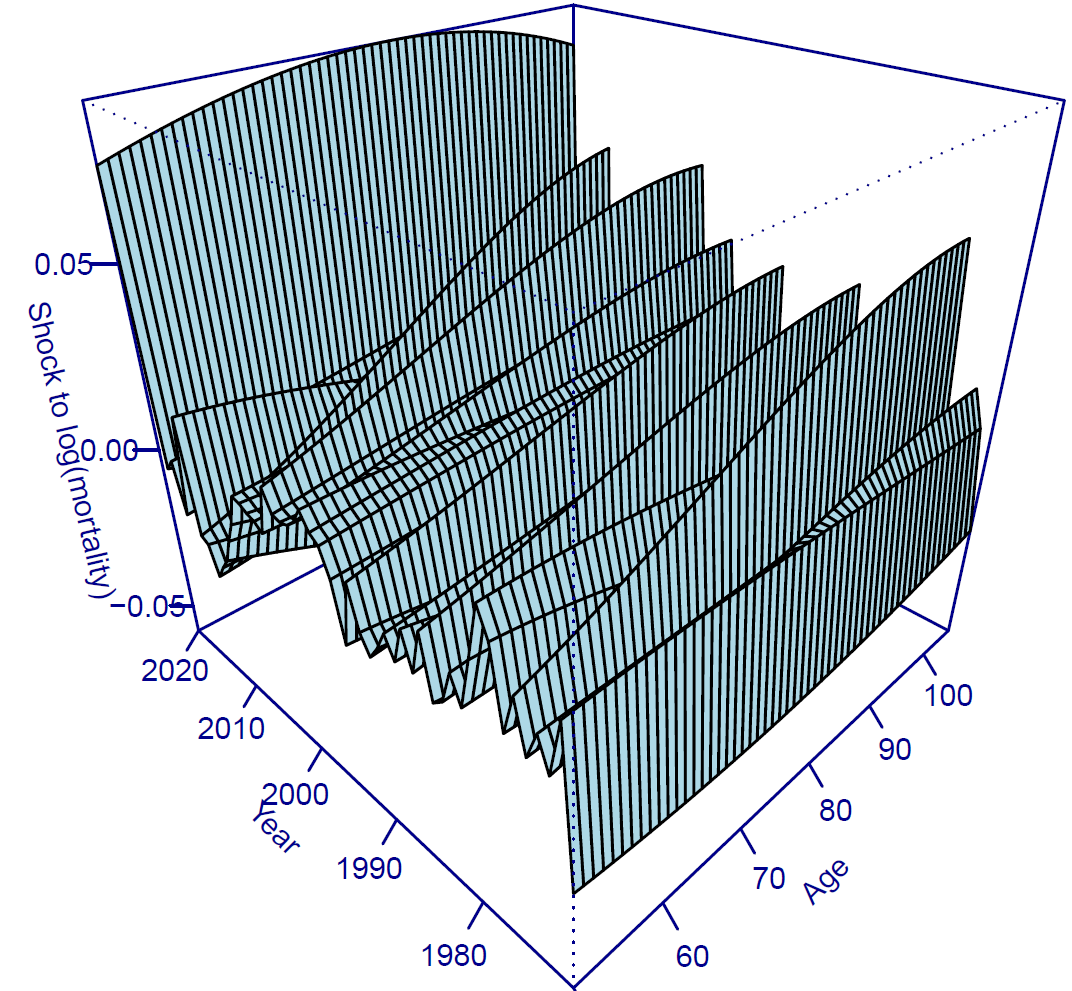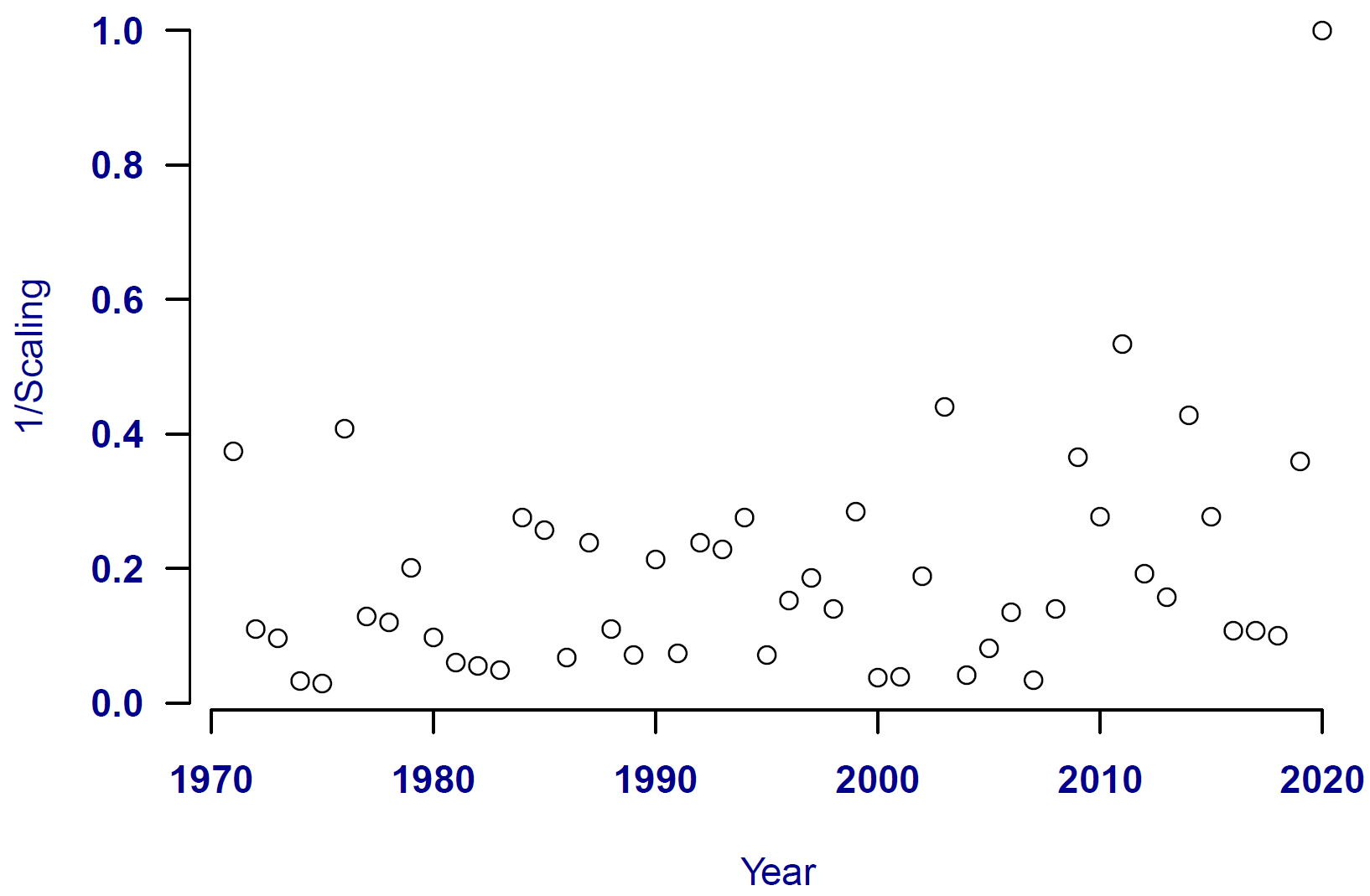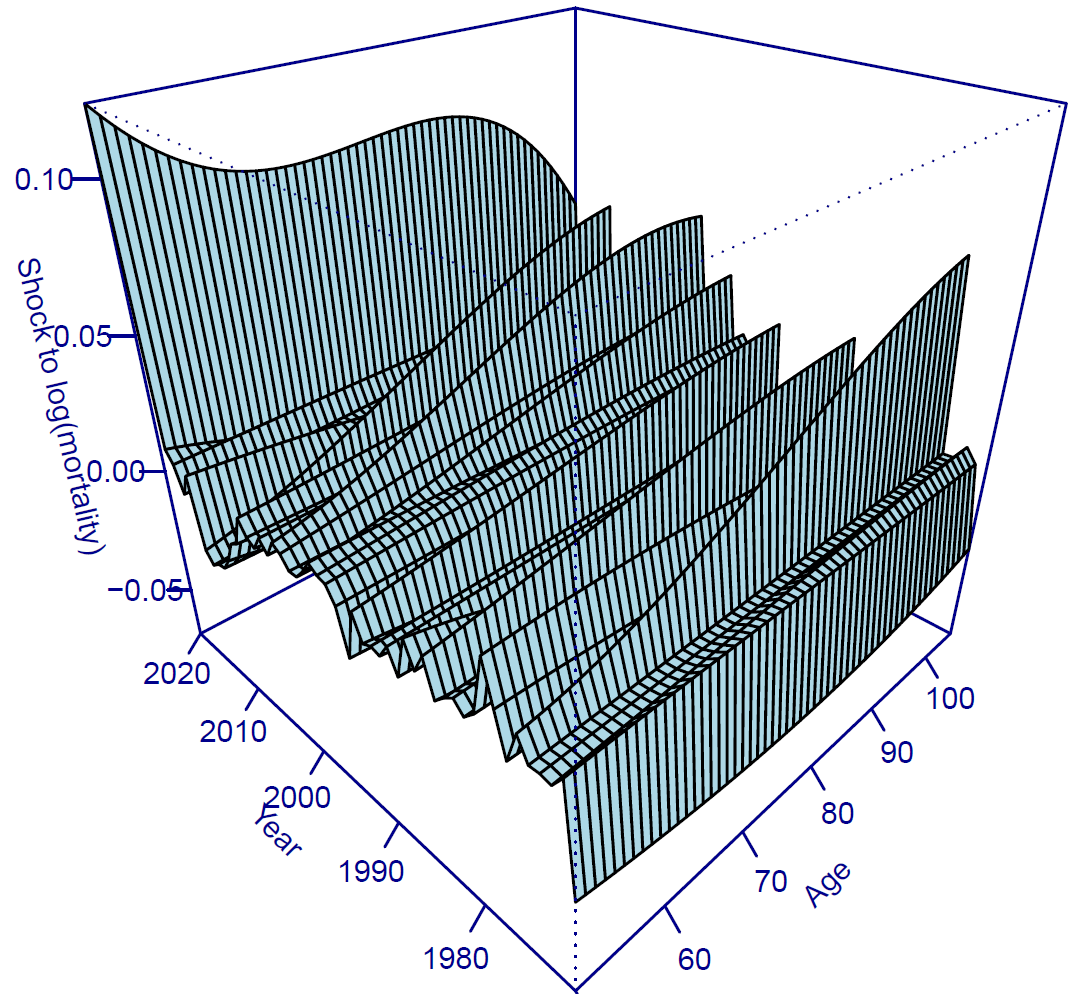# Robust mortality forecasting for 2D age-period models

The covid-19 pandemic caused mortality shocks in many countries, and these shocks severely impact the standard forecasting models used by actuaries.  I previously showed how to robustify time-series models with a univariate index (Lee-Carter, APC) and those with a multivariate index (Cairns-Blake-Dowd, Tang-Li-Tickle).  One model class not covered so far is the 2D P-spline model of Currie, Durban & Eilers (2004).

As it happens, the topic of mortality shocks in 2D models was addressed by Kirkby & Currie (2010); the authors specified a model with period shocks and applied it to Swedish mortality data including the 1918-1919 Spanish Influenza pandemic.  Iain Currie wrote an introductory blog on this topic as long ago as January 2009.  Here we will delve a little deeper into the technical details.

The 2D P-spline model is defined by Kirby & Currie (2010, equation 2.9) as a generalized linear array model (GLAM) as follows:

$\log \boldsymbol{M} = \log \boldsymbol{E}+\boldsymbol{B}_a\boldsymbol{\Theta}\boldsymbol{B}_y'\qquad(1)$

where

• $$\boldsymbol{M}$$ is the matrix of mortality rates indexed by age in the rows and by calendar year in the columns,
• $$\boldsymbol{E}$$ is the corresponding matrix of population exposures,
• $$\boldsymbol{B}_a$$ is a B-spline basis matrix for age,
• $$\boldsymbol{B}_y$$ is a B-spline basis matrix for time, and
• $$\boldsymbol{\Theta}$$ is a matrix of regression coefficients.

Fitting the GLAM is done by expressing equation (1) in vector form:

$\log {\rm vec}(\boldsymbol{M}) = \log {\rm vec}(\boldsymbol{E}) + (\boldsymbol{B}_y\otimes\boldsymbol{B}_a)\boldsymbol{\theta}\qquad(2)$

where the $${\rm vec}()$$ function stacks the columns in a matrix into a single vector, and where $$\boldsymbol{\theta}={\rm vec}(\boldsymbol{\Theta})$$.  In the fitting procedure, the coefficients in $$\boldsymbol{\Theta}$$ are simultaneously smoothed in the age and time dimensions by suitable penalty functions.  The problem with a mortality shock is that smoothing in the time dimension is no longer sensible.  Kirkby & Currie (2010) therefore extended the model in equation (2) to include period shocks as follows:

$\log {\rm vec}(\boldsymbol{M}) = \log {\rm vec}(\boldsymbol{E}) + (\boldsymbol{B}_y\otimes\boldsymbol{B}_a)\boldsymbol{\theta}+(\boldsymbol{I}_{n_y}\otimes\boldsymbol{\breve{B}}_s)\boldsymbol{\breve{\theta}}\qquad(3)$

where $$\boldsymbol{I}_{n_y}$$ is the identity matrix for $$n_y$$ years, $$\boldsymbol{\breve{B}}_s$$ is a B-spline basis in age and $$\boldsymbol{\breve{\theta}}$$ is a vector of shock coefficients.  $$\boldsymbol{\breve{B}}_s$$ and $$\boldsymbol{B}_a$$ are both basis matrices in age, but $$\boldsymbol{\breve{B}}_s$$ typically has fewer knots than $$\boldsymbol{B}_a$$.  When applied to the mortality of females in England & Wales, equation (3) reveals the period shocks shown in Figure 1:

Figure 1. Simple period shocks under model in equation (3).  Source: own calculations using data for females in England & Wales aged 50-105, over the period 1971-2020.Figure 1 shows that the model of Kirkby & Currie (2010) picks up the covid-19 mortality shock of 2020, but also various minor period shocks since 1971.  However, for robustification we are arguably less interested in the minor shocks and would prefer to smooth the most trivial ones to zero.  Kirkby & Currie (2010) therefore proposed an extension whereby the amount of smoothing varies according to a scaling factor, which in turn is defined by a given year's shock size relative to the largest shock.  Thus, if the basic smoothing parameter for all years is $$\lambda_s$$, the scaled smoothing parameter for year $$i$$, $$\lambda_i$$, is then:

$\lambda_i = \lambda_s\left(\frac{{\rm largest\ shock\ size}}{{\rm shock\ size\ for\ year\ }i}\right)^\alpha,\quad i=1,\ldots,n_y\qquad(4)$

By way of illustration, Figure 2 shows the inverse scaling factors ($$\lambda_s/\lambda_i$$) for simple scaling with $$\alpha=1$$; the years 1974 and 1975 receive more than ten times the smoothing than 2020, for example.

Figure 2.  Inverse of simple scaling factors ($$\lambda_s/\lambda_i$$) for 2D period-shock model of Kirkby & Currie (2010).  Source: own calculations using data for females in England & Wales aged 50-105, over the period 1971-2020.(The use of the inverse scaling factor makes Figure 2 vaguely analogous to the Mahalanobis distance in multivariate time-series robustification, albeit the two measures are very different.)

As it happens, equation (4) gives rise to a number of smoothing options:

• No scaling, i.e. $$\alpha=0$$ (as used in the model behind Figure 1),
• Simple scaling, i.e. $$\alpha=1$$ (as shown in Figure 2),
• Fixed scaling, i.e. $$\alpha$$ set to a given value, or
• Optimised scaling, i.e. $$\alpha$$ is set by minimising an information criterion such as the BIC.

Figure 3 shows the revised period shocks under optimised scaling, which shows that minor period effects are smoothed towards zero, leaving a focus on the most significant (and otherwise most distorting) shocks.  The result is that the true extent of the 2020 shock is revealed.

Figure 3.  Period shocks under optimised scaling using equation (4).  Source: own calculations using data for females in England & Wales aged 50-105, over the period 1971-2020.As with univariate and multivariate robustification, the use of the 2D period-shock model removes the distorting influence of the covid-19 pandemic.  This produces more sensible forecasts without the undue influence of outliers.

References:

Currie, I. D., Durban, M. and Eilers, P. H. C. (2004) Smoothing and forecasting mortality rates, Statistical Modelling, 4, 279-298.

Kirkby, J. G. and Currie, I. D. (2010) Smooth models of mortality with period shocks, Statistical Modelling, 10(2), 177-196.Written by: Stephen Richards
Publication Date:
Last Updated:

# Robust 2D forecasts

From v2.8.7 users of the Projection Toolkit can fit robustified 2D age-period P-spline models.  The robustification methodology is selected with the Fit 2D Shocks Model option, while the knot spacing for the period shocks is set by the 2D Shock Age Knot Spacing option.  The choice of whether to apply scaling to the shocks is controlled by the 2D Shock Scaling option

## Previous posts

### Unpoking the bear

The human immune system is a powerful defender, using its arsenal of antibodies to identify and attack foreign pathogens. But such antibodies have a potential dark side: autoantibodies that begin to attack, not invading microbes, but healthy tissues.
Tags: Filter information matrix by tag: immunotherapy

### M is for Estimation

In earlier blogs I discussed two techniques for handling outliers in mortality forecasting models:

Tags: Filter information matrix by tag: outliers, Filter information matrix by tag: robustness, Filter information matrix by tag: log-likelihood